# How to convert unstructured data to structured data using Python ?

• Last Updated : 21 Apr, 2022

Prerequisite: What is Unstructured Data?

Sometimes machine generates data in an unstructured way which is less interpretable. For example, Biometric Data, where an employee does Punch – IN or OUT several times with mistakes. We can not analyze the data and identify the mistakes unless it’s in a tabular form. In this article, we will take unstructured biometric data and convert it into useful information in terms of a table.

### Dataset:

Here we will work with Daily Punch – In Report. Data is given below. Punch records captured for Main Door and Second Door. Main Door is Outdoor Gate and Second Door is Project Room Gate. We need to identify which employee spent how much time in the project room or second door. The dataset we’re going to use is Bio.xlsx: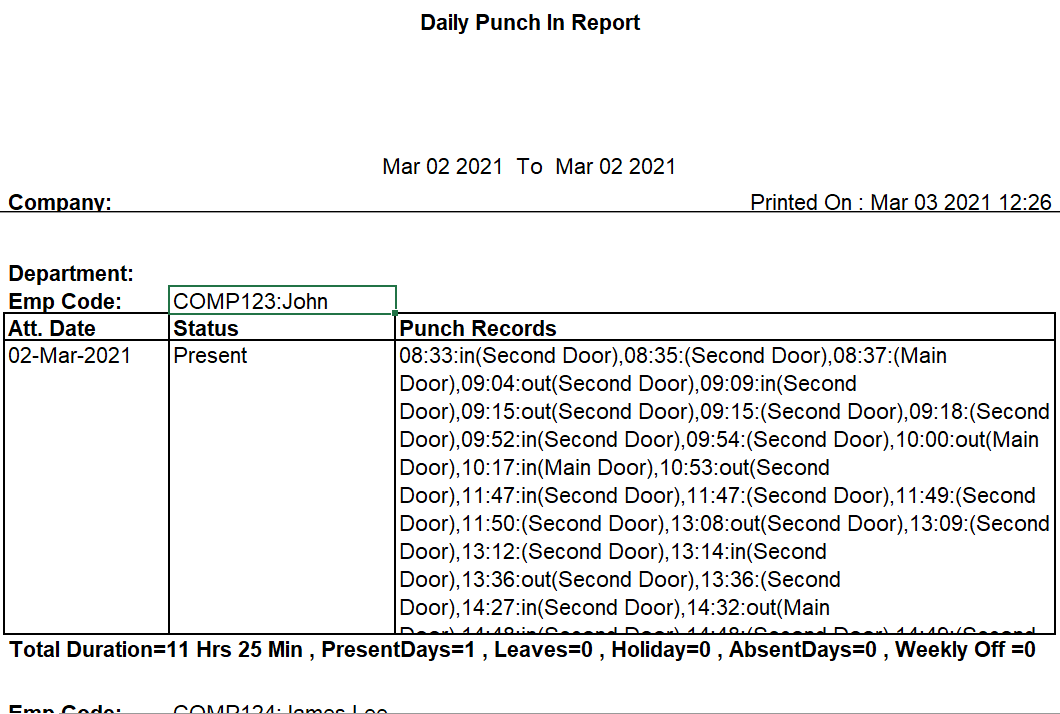Here is the Biometric Data for John Sherrif where punch in and punch out records has given for Main Door and Second Door.

The above Data is not insightful to analyze. Our desired output is:

Understanding the Data: John Sherrif did Punch – IN at 08:33 for the first time and Punch – OUT at 09:04 for the first time. John did Punch – IN at 14:27 but forgot to do Punch – OUT. The ‘in’ signifies he/she forgot to do Punch IN and ‘out’ signifies vice versa.

### Implementation:

• Data Cleaning & Creating a table for status, Punch Code, and Emp Code.

## Python3

 `import` `pandas as pd` `# load data``df ``=` `pd.read_excel(``'bio.xlsx'``)` `# removing NA values from the``# dataframe df``df ``=` `df.fillna("")` `# removing all the blank rows``df1 ``=` `df.dropna(how``=``'all'``)` `# picking the rows where present``# or absent values are there from``# 14 no column``df1 ``=` `df1[df1[``'Unnamed: 14'``].``str``.contains(``'sent'``)]` `# Extracting only the Employee``# Names``df_name ``=` `df.dropna(how``=``'all'``)` `# from column no 3 we are picking``# Employee names``df_name ``=` `df_name[df_name[``'Unnamed: 3'``].``str``.contains(``'Employee'``)]` `# creating a new dataframe for Status,``# Punch Records and Employee Codes``zippedList ``=` `list``(``    ``zip``(df1[``'Unnamed: 14'``], df1[``'Unnamed: 15'``], df_name[``'Unnamed: 7'``]))` `abc ``=` `pd.DataFrame(zippedList)``abc.head()`

Output: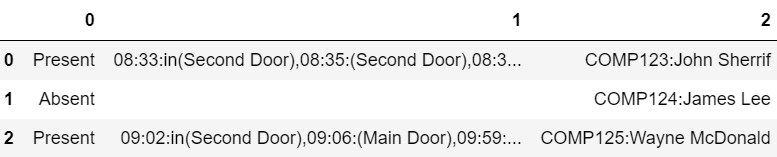• Extracting Data for Second Door only.

## Python3

 `# Splitting the values by comma in 1``# no column (punch records)``for` `i ``in` `range``(``len``(abc)):``    ``abc[``1``][i] ``=` `abc[``1``][i].split(``","``)` `second_door ``=` `[]` `for` `i ``in` `range``(``len``(abc)):``    ``s_d ``=` `[]``    ` `    ``# Extracting all the values which contains``    ``# only :in(Second Door) or :out(Second Dorr)``    ``for` `j ``in` `range``(``len``(abc[``1``][i])):``        ``if` `':in(Second Door)'` `in` `abc[``1``][i][j]:``            ``s_d.append(abc[``1``][i][j])` `        ``if` `'out(Second Door)'` `in` `abc[``1``][i][j]:``            ``s_d.append(abc[``1``][i][j])` `    ``second_door.append(s_d)``(second_door[``0``])`

Output: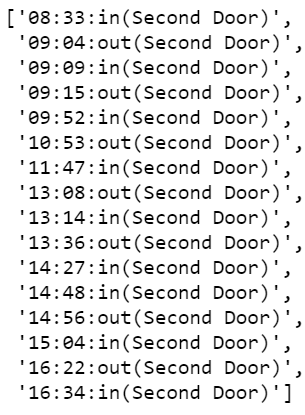• The punch record should start with ‘IN’ and end with ‘OUT’. Creating the pattern if it doesn’t follow.

## Python3

 `# Punch Records should start with``# the keyword 'in'. If it doesn't``# follow then we will add 'in' and it``# significants that the employee forgot``# to do punch in``in_time ``=` `[]``for` `i ``in` `range``(``len``(second_door)):``    ``try``:``        ``if` `':in(Second Door)'` `not` `in` `second_door[i][``0``]:``            ``second_door[i].insert(``0``, ``'in'``)` `    ``except``:``        ``pass``      ` `# Punch Records should end with the keyword``# 'out'. If it doesn't follow then we will``# add 'out' and it  significants that the``# employee forgot to do punch out``out_time ``=` `[]``for` `i ``in` `range``(``len``(second_door)):``    ``try``:` `        ``if` `':out(Second Door)'` `not` `in` `second_door[i][(``len``(second_door[i]))``-``1``]:``            ``second_door[i].insert(((``len``(second_door[i]))), ``'out'``)``    ``except``:``        ``pass``second_door[``0``]`

Output: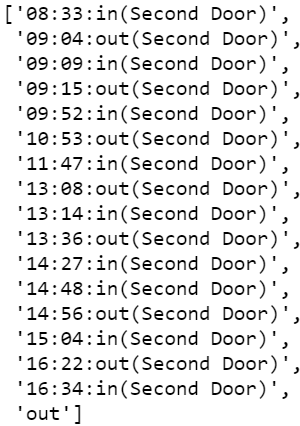• Creating the pattern ‘IN – OUT – IN – …..- OUT’. If someone forgot to do Punch – IN then we will put ‘IN’ & if someone forgot to do Punch – OUT then we will put ‘OUT’.

## Python3

 `# final_in contains PUNCH - IN``# records for all employees``final_in ``=` `[]` `# final_out contains PUNCH - OUT``# records for all employees``final_out ``=` `[]` `for` `k ``in` `range``(``len``(second_door)):``    ``in_gate ``=` `[]``    ``out_gate ``=` `[]``    ` `    ``# even position should be for Punch-``    ``# IN and odd position should be for``    ``# Punch - OUT if it doesn't follow``    ``# then we will create the pattern by``    ``# putting 'in' or 'out'``    ``for` `i ``in` `range``(``len``(second_door[k])):``        ``if` `i ``%` `2` `=``=` `0` `and` `'in'` `in` `second_door[k][i]:``            ``in_gate.append(second_door[k][i])``            ``try``:``                ``if` `'out'` `not` `in` `second_door[k][i``+``1``]:``                    ``out_gate.append(``'out'``)``            ``except``:``                ``pass``        ``if` `i ``%` `2` `!``=` `0` `and` `'out'` `in` `second_door[k][i]:``            ``out_gate.append(second_door[k][i])``            ``try``:``                ``if` `'in'` `not` `in` `second_door[k][i``+``1``]:``                    ``in_gate.append(``'in'``)``            ``except``:``                ``pass``        ``if` `i ``%` `2` `!``=` `0` `and` `'in'` `in` `second_door[k][i]:``            ``in_gate.append(second_door[k][i])` `            ``try``:``                ``if` `'out'` `not` `in` `second_door[k][i``+``1``]:``                    ``out_gate.append(``'out'``)``            ``except``:``                ``pass` `        ``if` `i ``%` `2` `=``=` `0` `and` `'out'` `in` `second_door[k][i]:``            ``out_gate.append(second_door[k][i])` `            ``try``:``                ``if` `'in'` `not` `in` `second_door[k][i``+``1``]:``                    ``in_gate.append(``'in'``)``            ``except``:``                ``pass``    ``final_in.append(in_gate)``    ``final_out.append(out_gate)` `# final_in or final_out keep the``# records as a list under list form.``# to solve the problem we will merge the list` `# aa contains merged list of Punch - IN``aa ``=` `final_in[``0``]``for` `i ``in` `range``(``len``(final_in)``-``1``):``    ``aa ``=` `aa ``+` `final_in[i``+``1``]``    ` `# bb contains merged list of Punch - OUT``bb ``=` `final_out[``0``]``for` `i ``in` `range``(``len``(final_out)``-``1``):``    ``bb ``=` `bb ``+` `final_out[i``+``1``]` `for` `i ``in` `range``(``len``(final_in[``0``])):``    ``print``(final_in[``0``][i], ``'   '``, final_out[``0``][i])`

Output: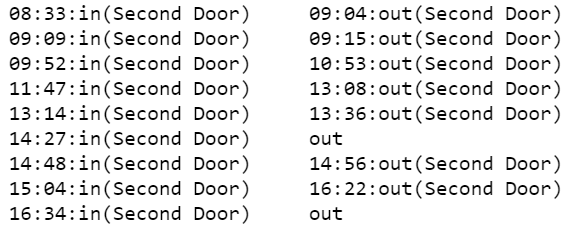• Creating the final table.

## Python

 `# Creating a dataframe called df_final``df_final ``=` `[]``df_final ``=` `pd.DataFrame(df_final)` `# Merging the Employee Names``Name ``=` `[]``for` `i ``in` `range``(``len``(abc)):``    ``for` `j ``in` `range``(``len``(final_in[i])):``        ``Name.append(abc[``2``][i])``df_final[``'Name'``] ``=` `Name` `# Zipping the Employee Name, Punch -IN``# records and Punch - OUT records``zippedList2 ``=` `list``(``zip``(df_final[``'Name'``], aa, bb))``abc2 ``=` `pd.DataFrame(zippedList2)` `# Renaming the dataframe``abc2.columns ``=` `[``'Emp Code'``, ``'Punch - IN'``, ``'Punch - OUT'``]``abc2.to_excel(``'output.xlsx'``, index``=``False``)` `# Print the table``display(abc2)`

Output: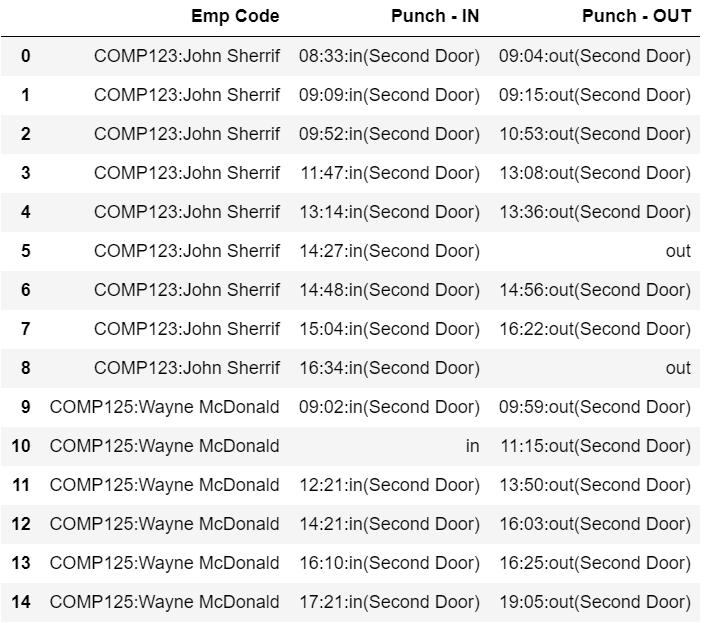Hence, the raw biometric data has been structured and is converted to useful information.

My Personal Notes arrow_drop_up